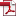• KSII Transactions on Internet and Information Systems
Monthly Online Journal (eISSN: 1976-7277)

# Achievable Sum Rate Analysis of ZF Receivers in 3D MIMO Systems

Vol. 8, No. 4, April 28, 201410.3837/tiis.2014.04.012, Download Paper (Free):#### Abstract

Three-dimensional multiple-input multiple-output (3D MIMO) and large-scale MIMO are two promising technologies for upcoming high data rate wireless communications, since the inter-user interference can be reduced by exploiting antenna vertical gain and degree of freedom, respectively. In this paper, we derive the achievable sum rate of 3D MIMO systems employing zero-forcing (ZF) receivers, accounting for log-normal shadowing fading, path-loss and antenna gain. In particular, we consider the prevalent log-normal model and propose a novel closed-form lower bound on the achievable sum rate exploiting elevation features. Using the lower bound as a starting point, we pursue the "large-system" analysis and derive a closed-form expression when the number of antennas grows large for fixed average transmit power and fixed total transmit power schemes. We further model a high-building with several floors. Due to the floor height, different floors correspond to different elevation angles. Therefore, the asymptotic achievable sum rate performances for each floor and the whole building considering the elevation features are analyzed and the effects of tilt angle and user distribution for both horizontal and vertical dimensions are discussed. Finally, the relationship between the achievable sum rate and the number of users is investigated and the optimal number of users to maximize the sum rate performance is determined.